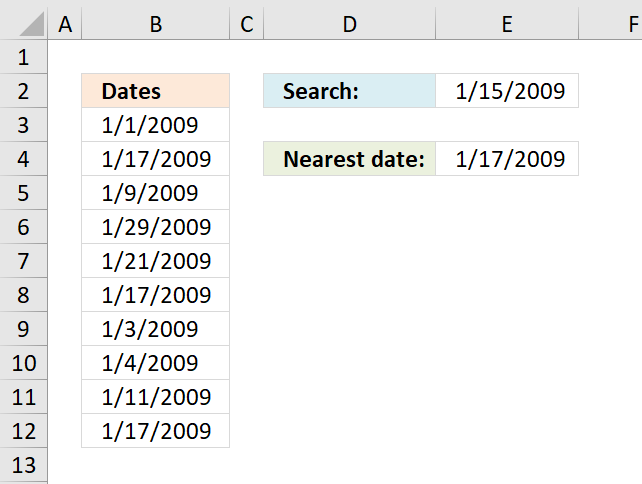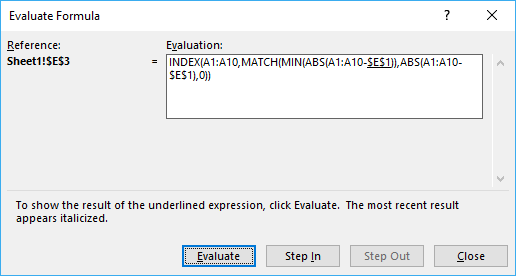Author: Oscar Cronquist Article last updated on July 08, 2018The image above demonstrates an array formula in cell E4 that searches for the closest date in column A to the date in cell E2.

Array formula in E4:

=INDEX(B3:B12, MATCH(MIN(ABS(B3:B12-\$E\$2)), ABS(B3:B12-\$E\$2), 0))

Recommended articles:

Find date range based on a date

This article demonstrates a formula that returns a date range that a date falls under, cell C3 above contains the […]

Formula for matching a date within a date range

This article demonstrates how to match a specified date to date ranges. The image above shows a formula in cell […]

### How to enter an array formula

1. Select cell E4
2. Type or copy/paste above array formula to formula bar3. Press and hold Ctrl + Shift
4. Press Enter

A beginners guide to Excel array formulas

Array formulas allows you to do advanced calculations not possible with regular formulas.

### Explaining array formula in cell E4

You can easily follow along, select cell E3. Go to tab "Formulas" and press with left mouse button on "Evaluate formula".Press with left mouse button on "Evaluate" button to move to next step.

#### Step 1 - Subtract dates with search date

B3:B12-\$E\$2

becomes

{39814; 39830; 39822; 39842; 39834; 39830; 39816; 39817; 39824; 39830}-39828

and returns {-14;2;-6;14;6;2;-12;-11;-4;2}

#### Step 2 - Convert numerical values to absolute values

The ABS function converts a negative number to a positive.

ABS(B3:B12-\$E\$2)

becomes

ABS({-14;2;-6;14;6;2;-12;-11;-4;2})

and returns {14;2;6;14;6;2;12;11;4;2}

#### Step 3 - Find smallest numerical value in array

The MIN function returns the smallest number in a cell range or array.

MIN(ABS(B3:B12-\$E\$2))

becomes

MIN({14;2;6;14;6;2;12;11;4;2})

and returns 2.

#### Step 4 - Find position in array

The MATCH function returns the relative position of a given value in an array or cell range.

MATCH(MIN(ABS(B3:B12-\$E\$2)), ABS(B3:B12-\$E\$2), 0)

becomes

MATCH(2, ABS(B3:B12-\$E\$2), 0)

becomes

MATCH(2, {14;2;6;14;6;2;12;11;4;2}, 0)

and returns 2. Numerical value 2 has position 2 in the array.

How to use the MATCH function

Identify the position of a value in an array.

#### Step 5 - Return value

INDEX(B3:B12, MATCH(MIN(ABS(B3:B12-\$E\$2)), ABS(B3:B12-\$E\$2), 0))

becomes

INDEX(B3:B12, 2)

becomes

INDEX({39814;39830;39822; 39842;39834;39830; 39816;39817;39824;39830}, 2)

an returns 39830 or 1-17-2009 in cell E4.

How to use the INDEX function

Gets a value in a specific cell range based on a row and column number.

### Get excel example file

find-nearest-date.xls
(Excel 97-2003 Workbook *.xls)

Recommended articles:

Lookup min max values within a date range

This article explains how to find the smallest and largest value using two conditions. In this case they are date […]

Find latest date based on a condition

This article demonstrates how to return the latest date based on a condition using formulas or a Pivot Table. The […]